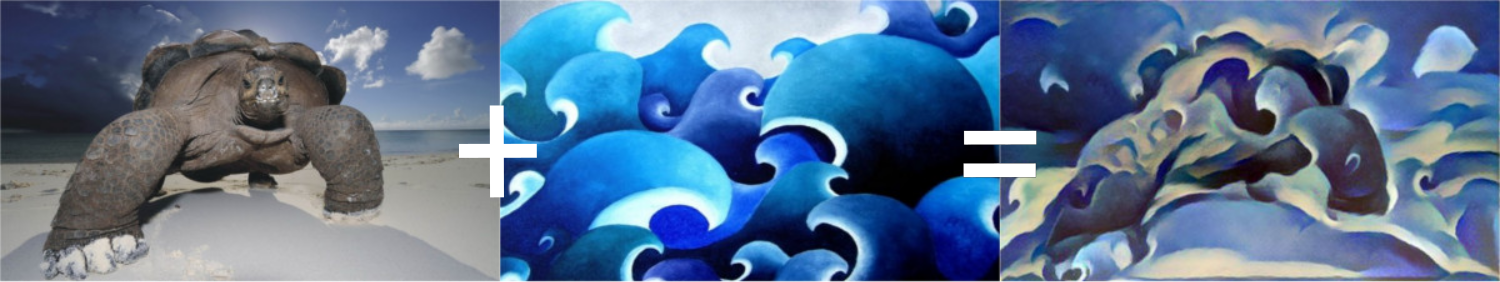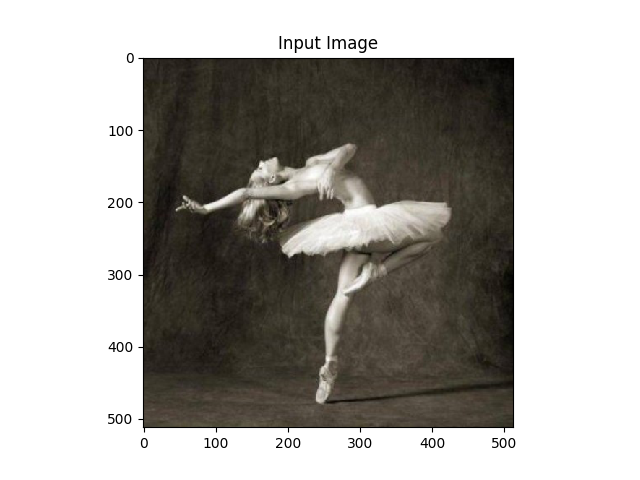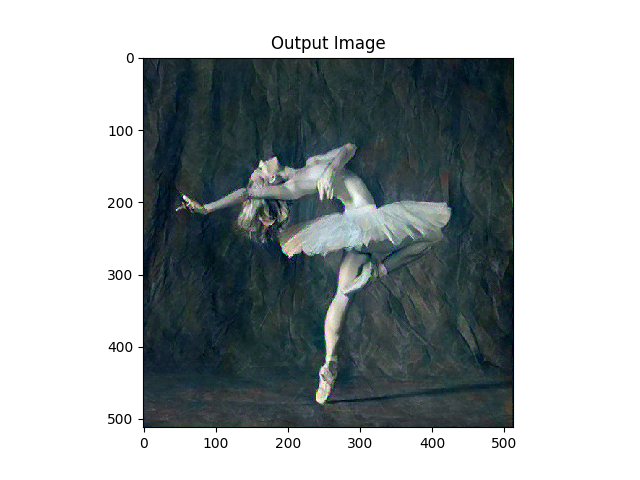# Neural Transfer with PyTorch¶

Author: Alexis Jacq

## Introduction¶

Welcome! This tutorial explains how to impletment the Neural-Style algorithm developed by Leon A. Gatys, Alexander S. Ecker and Matthias Bethge.

### Neural what?¶

The Neural-Style, or Neural-Transfer, is an algorithm that takes as input a content-image (e.g. a tortle), a style-image (e.g. artistic waves) and return the content of the content-image as if it was ‘painted’ using the artistic style of the style-image:### How does it work?¶

The principle is simple: we define two distances, one for the content ($$D_C$$) and one for the style ($$D_S$$). $$D_C$$ measures how different the content is between two images, while $$D_S$$ measures how different the style is between two images. Then, we take a third image, the input, (e.g. a with noise), and we transform it in order to both minimize its content-distance with the content-image and its style-distance with the style-image.

#### OK. How does it work?¶

Well, going further requires some mathematics. Let $$C_{nn}$$ be a pre-trained deep convolutional neural network and $$X$$ be any image. $$C_{nn}(X)$$ is the network fed by $$X$$ (containing feature maps at all layers). Let $$F_{XL} \in C_{nn}(X)$$ be the feature maps at depth layer $$L$$, all vectorized and concatenated in one single vector. We simply define the content of $$X$$ at layer $$L$$ by $$F_{XL}$$. Then, if $$Y$$ is another image of same the size than $$X$$, we define the distance of content at layer $$L$$ as follow:

$D_C^L(X,Y) = \|F_{XL} - F_{YL}\|^2 = \sum_i (F_{XL}(i) - F_{YL}(i))^2$

Where $$F_{XL}(i)$$ is the $$i^{th}$$ element of $$F_{XL}$$. The style is a bit less trivial to define. Let $$F_{XL}^k$$ with $$k \leq K$$ be the vectorized $$k^{th}$$ of the $$K$$ feature maps at layer $$L$$. The style $$G_{XL}$$ of $$X$$ at layer $$L$$ is defined by the Gram produce of all vectorized feature maps $$F_{XL}^k$$ with $$k \leq K$$. In other words, $$G_{XL}$$ is a $$K$$x$$K$$ matrix and the element $$G_{XL}(k,l)$$ at the $$k^{th}$$ line and $$l^{th}$$ column of $$G_{XL}$$ is the vectorial produce between $$F_{XL}^k$$ and $$F_{XL}^l$$ :

$G_{XL}(k,l) = \langle F_{XL}^k, F_{XL}^l\rangle = \sum_i F_{XL}^k(i) . F_{XL}^l(i)$

Where $$F_{XL}^k(i)$$ is the $$i^{th}$$ element of $$F_{XL}^k$$. We can see $$G_{XL}(k,l)$$ as a measure of the correlation between feature maps $$k$$ and $$l$$. In that way, $$G_{XL}$$ represents the correlation matrix of feature maps of $$X$$ at layer $$L$$. Note that the size of $$G_{XL}$$ only depends on the number of feature maps, not on the size of $$X$$. Then, if $$Y$$ is another image of any size, we define the distance of style at layer $$L$$ as follow:

$D_S^L(X,Y) = \|G_{XL} - G_{YL}\|^2 = \sum_{k,l} (G_{XL}(k,l) - G_{YL}(k,l))^2$

In order to minimize in one shot $$D_C(X,C)$$ between a variable image $$X$$ and target content-image $$C$$ and $$D_S(X,S)$$ between $$X$$ and target style-image $$S$$, both computed at several layers , we compute and sum the gradients (derivative with respect to $$X$$) of each distance at each wanted layer:

$\nabla_{ extit{total}}(X,S,C) = \sum_{L_C} w_{CL_C}.\nabla_{ extit{content}}^{L_C}(X,C) + \sum_{L_S} w_{SL_S}.\nabla_{ extit{style}}^{L_S}(X,S)$

Where $$L_C$$ and $$L_S$$ are respectivement the wanted layers (arbitrary stated) of content and style and $$w_{CL_C}$$ and $$w_{SL_S}$$ the weights (arbitrary stated) associated with the style or the content at each wanted layer. Then, we run a gradient descent over $$X$$:

$X \leftarrow X - \alpha \nabla_{ extit{total}}(X,S,C)$

Ok. That’s enough with maths. If you want to go deeper (how to compute the gradients) we encourage you to read the original paper by Leon A. Gatys and AL, where everything is much better and much clearer explained.

For our implementation in PyTorch, we already have everything we need: indeed, with PyTorch, all the gradients are automatically and dynamically computed for you (while you use functions from the library). This is why the implementation of this algorithm becomes very comfortable with PyTorch.

## PyTorch implementation¶

If you are not sure to understand all the mathematics above, you will probably get it by implementing it. If you are discovering PyTorch, we recommend you to first read this Introduction to PyTorch.

### Packages¶

We will have recourse to the following packages:

• torch, torch.nn, numpy (indispensables packages for neural networks with PyTorch)
• torch.autograd.Variable (dynamic computation of the gradient wrt a variable)
• torch.optim (efficient gradient descents)
• PIL, PIL.Image, matplotlib.pyplot (load and display images)
• torchvision.transforms (treat PIL images and transform into torch tensors)
• torchvision.models (train or load pre-trained models)
• copy (to deep copy the models; system package)
from __future__ import print_function

import torch
import torch.nn as nn
from torch.autograd import Variable
import torch.optim as optim

from PIL import Image
import matplotlib.pyplot as plt

import torchvision.transforms as transforms
import torchvision.models as models

import copy


### Cuda¶

If you have a GPU on your computer, it is preferable to run the algorithm on it, especially if you want to try larger networks (like VGG). For this, we have torch.cuda.is_available() that returns True if you computer has an available GPU. Then, we can use method .cuda() that moves allocated proccesses associated with a module from the CPU to the GPU. When we want to move back this module to the CPU (e.g. to use numpy), we use the .cpu() method. Finally, .type(dtype) will be use to convert a torch.FloatTensor into torch.cuda.FloatTensor to feed GPU processes.

use_cuda = torch.cuda.is_available()
dtype = torch.cuda.FloatTensor if use_cuda else torch.FloatTensor


### Load images¶

In order to simplify the implementation, let’s start by importing a style and a content image of the same dimentions. We then scale them to the desired output image size (128 or 512 in the example, depending on gpu availablity) and transform them into torch tensors, ready to feed a neural network:

Note

Here are links to download the images required to run the tutorial: picasso.jpg and dancing.jpg. Download these two images and add them to a directory with name images

# desired size of the output image
imsize = 512 if use_cuda else 128  # use small size if no gpu

loader = transforms.Compose([
transforms.Scale(imsize),  # scale imported image
transforms.ToTensor()])  # transform it into a torch tensor

def image_loader(image_name):
image = Image.open(image_name)
image = Variable(loader(image))
# fake batch dimension required to fit network's input dimensions
image = image.unsqueeze(0)
return image

style_img = image_loader("images/picasso.jpg").type(dtype)
content_img = image_loader("images/dancing.jpg").type(dtype)

assert style_img.size() == content_img.size(), \
"we need to import style and content images of the same size"


Imported PIL images has values between 0 and 255. Transformed into torch tensors, their values are between 0 and 1. This is an important detail: neural networks from torch library are trained with 0-1 tensor image. If you try to feed the networks with 0-255 tensor images the activated feature maps will have no sense. This is not the case with pre-trained networks from the Caffe library: they are trained with 0-255 tensor images.

### Display images¶

We will use plt.imshow to display images. So we need to first reconvert them into PIL images:

unloader = transforms.ToPILImage()  # reconvert into PIL image

plt.ion()

def imshow(tensor, title=None):
image = tensor.clone().cpu()  # we clone the tensor to not do changes on it
image = image.view(3, imsize, imsize)  # remove the fake batch dimension
image = unloader(image)
plt.imshow(image)
if title is not None:
plt.title(title)
plt.pause(0.001) # pause a bit so that plots are updated

plt.figure()
imshow(style_img.data, title='Style Image')

plt.figure()
imshow(content_img.data, title='Content Image')


### Content loss¶

The content loss is a function that takes as input the feature maps $$F_{XL}$$ at a layer $$L$$ in a network fed by $$X$$ and return the weigthed content distance $$w_{CL}.D_C^L(X,C)$$ between this image and the content image. Hence, the weight $$w_{CL}$$ and the target content $$F_{CL}$$ are parameters of the function. We implement this function as a torch module with a constructor that takes these parameters as input. The distance $$\|F_{XL} - F_{YL}\|^2$$ is the Mean Square Error between the two sets of feature maps, that can be computed using a criterion nn.MSELoss stated as a third parameter.

We will add our content losses at each desired layer as additive modules of the neural network. That way, each time we will feed the network with an input image $$X$$, all the content losses will be computed at the desired layers and, thanks to autograd, all the gradients will be computed. For that, we just need to make the forward method of our module returning the input: the module becomes a ‘’transparent layer’’ of the neural network. The computed loss is saved as a parameter of the module.

Finally, we define a fake backward method, that just call the backward method of nn.MSELoss in order to reconstruct the gradient. This method returns the computed loss: this will be useful when running the gradient descent in order to display the evolution of style and content losses.

class ContentLoss(nn.Module):

def __init__(self, target, weight):
super(ContentLoss, self).__init__()
# we 'detach' the target content from the tree used
self.target = target.detach() * weight
# to dynamically compute the gradient: this is a stated value,
# not a variable. Otherwise the forward method of the criterion
# will throw an error.
self.weight = weight
self.criterion = nn.MSELoss()

def forward(self, input):
self.loss = self.criterion(input * self.weight, self.target)
self.output = input
return self.output

def backward(self, retain_graph=True):
self.loss.backward(retain_graph=retain_graph)
return self.loss


Note

Important detail: this module, although it is named ContentLoss, is not a true PyTorch Loss function. If you want to define your content loss as a PyTorch Loss, you have to create a PyTorch autograd Function and to recompute/implement the gradient by the hand in the backward method.

### Style loss¶

For the style loss, we need first to define a module that compute the gram produce $$G_{XL}$$ given the feature maps $$F_{XL}$$ of the neural network fed by $$X$$, at layer $$L$$. Let $$\hat{F}_{XL}$$ be the re-shaped version of $$F_{XL}$$ into a $$K$$x$$N$$ matrix, where $$K$$ is the number of feature maps at layer $$L$$ and $$N$$ the lenght of any vectorized feature map $$F_{XL}^k$$. The $$k^{th}$$ line of $$\hat{F}_{XL}$$ is $$F_{XL}^k$$. We let you check that $$\hat{F}_{XL} \cdot \hat{F}_{XL}^T = G_{XL}$$. Given that, it becomes easy to implement our module:

class GramMatrix(nn.Module):

def forward(self, input):
a, b, c, d = input.size()  # a=batch size(=1)
# b=number of feature maps
# (c,d)=dimensions of a f. map (N=c*d)

features = input.view(a * b, c * d)  # resise F_XL into \hat F_XL

G = torch.mm(features, features.t())  # compute the gram product

# we 'normalize' the values of the gram matrix
# by dividing by the number of element in each feature maps.
return G.div(a * b * c * d)


The longer is the feature maps dimension $$N$$, the bigger are the values of the gram matrix. Therefore, if we don’t normalize by $$N$$, the loss computed at the first layers (before pooling layers) will have much more importance during the gradient descent. We dont want that, since the most interesting style features are in the deepest layers!

Then, the style loss module is implemented exactly the same way than the content loss module, but we have to add the gramMatrix as a parameter:

class StyleLoss(nn.Module):

def __init__(self, target, weight):
super(StyleLoss, self).__init__()
self.target = target.detach() * weight
self.weight = weight
self.gram = GramMatrix()
self.criterion = nn.MSELoss()

def forward(self, input):
self.output = input.clone()
self.G = self.gram(input)
self.G.mul_(self.weight)
self.loss = self.criterion(self.G, self.target)
return self.output

def backward(self, retain_graph=True):
self.loss.backward(retain_graph=retain_graph)
return self.loss


### Load the neural network¶

Now, we have to import a pre-trained neural network. As in the paper, we are going to use a pretrained VGG network with 19 layers (VGG19).

PyTorch’s implementation of VGG is a module divided in two child Sequential modules: features (containing convolution and pooling layers) and classifier (containing fully connected layers). We are just interested by features:

cnn = models.vgg19(pretrained=True).features

# move it to the GPU if possible:
if use_cuda:
cnn = cnn.cuda()


A Sequential module contains an ordered list of child modules. For instance, vgg19.features contains a sequence (Conv2d, ReLU, Maxpool2d, Conv2d, ReLU…) aligned in the right order of depth. As we said in Content loss section, we wand to add our style and content loss modules as additive ‘transparent’ layers in our network, at desired depths. For that, we construct a new Sequential module, in wich we are going to add modules from vgg19 and our loss modules in the right order:

# desired depth layers to compute style/content losses :
content_layers_default = ['conv_4']
style_layers_default = ['conv_1', 'conv_2', 'conv_3', 'conv_4', 'conv_5']

def get_style_model_and_losses(cnn, style_img, content_img,
style_weight=1000, content_weight=1,
content_layers=content_layers_default,
style_layers=style_layers_default):
cnn = copy.deepcopy(cnn)

# just in order to have an iterable access to or list of content/syle
# losses
content_losses = []
style_losses = []

model = nn.Sequential()  # the new Sequential module network
gram = GramMatrix()  # we need a gram module in order to compute style targets

# move these modules to the GPU if possible:
if use_cuda:
model = model.cuda()
gram = gram.cuda()

i = 1
for layer in list(cnn):
if isinstance(layer, nn.Conv2d):
name = "conv_" + str(i)
model.add_module(name, layer)

if name in content_layers:
# add content loss:
target = model(content_img).clone()
content_loss = ContentLoss(target, content_weight)
model.add_module("content_loss_" + str(i), content_loss)
content_losses.append(content_loss)

if name in style_layers:
# add style loss:
target_feature = model(style_img).clone()
target_feature_gram = gram(target_feature)
style_loss = StyleLoss(target_feature_gram, style_weight)
model.add_module("style_loss_" + str(i), style_loss)
style_losses.append(style_loss)

if isinstance(layer, nn.ReLU):
name = "relu_" + str(i)
model.add_module(name, layer)

if name in content_layers:
# add content loss:
target = model(content_img).clone()
content_loss = ContentLoss(target, content_weight)
model.add_module("content_loss_" + str(i), content_loss)
content_losses.append(content_loss)

if name in style_layers:
# add style loss:
target_feature = model(style_img).clone()
target_feature_gram = gram(target_feature)
style_loss = StyleLoss(target_feature_gram, style_weight)
model.add_module("style_loss_" + str(i), style_loss)
style_losses.append(style_loss)

i += 1

if isinstance(layer, nn.MaxPool2d):
name = "pool_" + str(i)
model.add_module(name, layer)  # ***

return model, style_losses, content_losses


Note

In the paper they recommend to change max pooling layers into average pooling. With AlexNet, that is a small network compared to VGG19 used in the paper, we are not going to see any difference of quality in the result. However, you can use these lines instead if you want to do this substitution:

# avgpool = nn.AvgPool2d(kernel_size=layer.kernel_size,
#                         stride=layer.stride, padding = layer.padding)
# model.add_module(name,avgpool)


### Input image¶

Again, in order to simplify the code, we take an image of the same dimensions than content and style images. This image can be a white noise, or it can also be a copy of the content-image.

input_img = content_img.clone()
# if you want to use a white noise instead uncomment the below line:
# input_img = Variable(torch.randn(content_img.data.size())).type(dtype)

# add the original input image to the figure:
plt.figure()
imshow(input_img.data, title='Input Image')### Gradient descent¶

As Leon Gatys, the author of the algorithm, suggested here, we will use L-BFGS algorithm to run our gradient descent. Unlike training a network, we want to train the input image in order to minimise the content/style losses. We would like to simply create a PyTorch L-BFGS optimizer, passing our image as the variable to optimize. But optim.LBFGS takes as first argument a list of PyTorch Variable that require gradient. Our input image is a Variable but is not a leaf of the tree that requires computation of gradients. In order to show that this variable requires a gradient, a possibility is to construct a Parameter object from the input image. Then, we just give a list containing this Parameter to the optimizer’s constructor:

def get_input_param_optimizer(input_img):
# this line to show that input is a parameter that requires a gradient
input_param = nn.Parameter(input_img.data)
optimizer = optim.LBFGS([input_param])
return input_param, optimizer


Last step: the loop of gradient descent. At each step, we must feed the network with the updated input in order to compute the new losses, we must run the backward methods of each loss to dynamically compute their gradients and perform the step of gradient descent. The optimizer requires as argument a “closure”: a function that reevaluates the model and returns the loss.

However, there’s a small catch. The optimized image may take its values between $$-\infty$$ and $$+\infty$$ instead of staying between 0 and 1. In other words, the image might be well optimized and have absurd values. In fact, we must perform an optimization under constraints in order to keep having right vaues into our input image. There is a simple solution: at each step, to correct the image to maintain its values into the 0-1 interval.

def run_style_transfer(cnn, content_img, style_img, input_img, num_steps=300,
style_weight=1000, content_weight=1):
"""Run the style transfer."""
print('Building the style transfer model..')
model, style_losses, content_losses = get_style_model_and_losses(cnn,
style_img, content_img, style_weight, content_weight)
input_param, optimizer = get_input_param_optimizer(input_img)

print('Optimizing..')
run = 
while run <= num_steps:

def closure():
# correct the values of updated input image
input_param.data.clamp_(0, 1)

optimizer.zero_grad()
model(input_param)
style_score = 0
content_score = 0

for sl in style_losses:
style_score += sl.backward()
for cl in content_losses:
content_score += cl.backward()

run += 1
if run % 50 == 0:
print("run {}:".format(run))
print('Style Loss : {:4f} Content Loss: {:4f}'.format(
style_score.data, content_score.data))
print()

return style_score + content_score

optimizer.step(closure)

# a last correction...
input_param.data.clamp_(0, 1)

return input_param.data


Finally, run the algorithm

output = run_style_transfer(cnn, content_img, style_img, input_img)

plt.figure()
imshow(output, title='Output Image')

# sphinx_gallery_thumbnail_number = 4
plt.ioff()
plt.show()Out:

Building the style transfer model..
Optimizing..
run :
Style Loss : 0.147848 Content Loss: 0.470452

run :
Style Loss : 0.043477 Content Loss: 0.343297

run :
Style Loss : 0.035841 Content Loss: 0.315429

run :
Style Loss : 0.031865 Content Loss: 0.306764

run :
Style Loss : 0.030837 Content Loss: 0.302101

run :
Style Loss : 0.030777 Content Loss: 0.299595


Total running time of the script: ( 1 minutes 10.112 seconds)

Gallery generated by Sphinx-Gallery